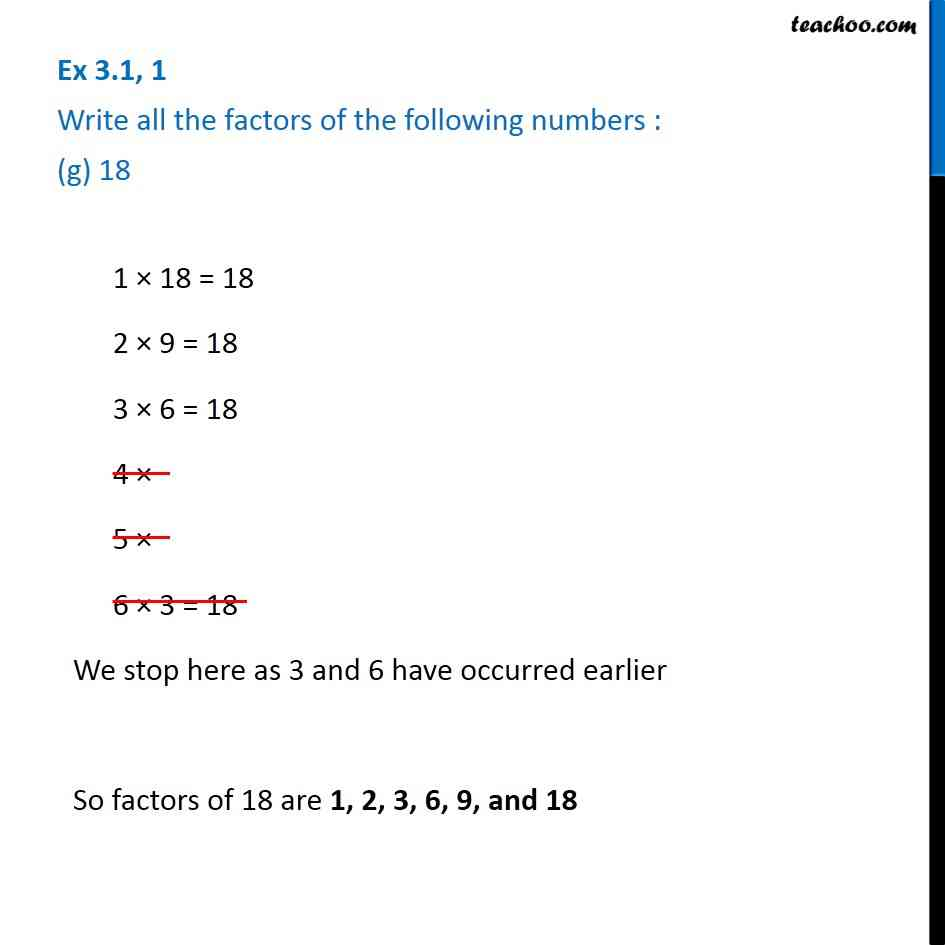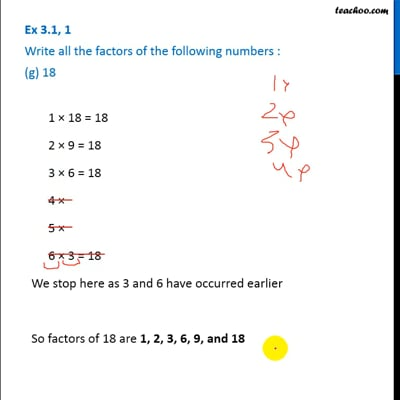Ex 3.1

Chapter 3 Class 6 Playing with Numbers
Serial order wiseThis video is only available for Teachoo black users

Introducing your new favourite teacher - Teachoo Black, at only ₹83 per month

### Transcript

Ex 3.1, 1 Write all the factors of the following numbers : (g) 18 1 × 18 = 18 2 × 9 = 18 3 × 6 = 18 4 × 5 × 6 × 3 = 18 We stop here as 3 and 6 have occurred earlier So factors of 18 are 1, 2, 3, 6, 9, and 18 1 × 18 = 18 2 × 9 = 18 3 × 6 = 18 4 × 5 × 6 × 3 = 18 We stop here as 3 and 6 have occurred earlier So factors of 18 are 1, 2, 3, 6, 9, and 18